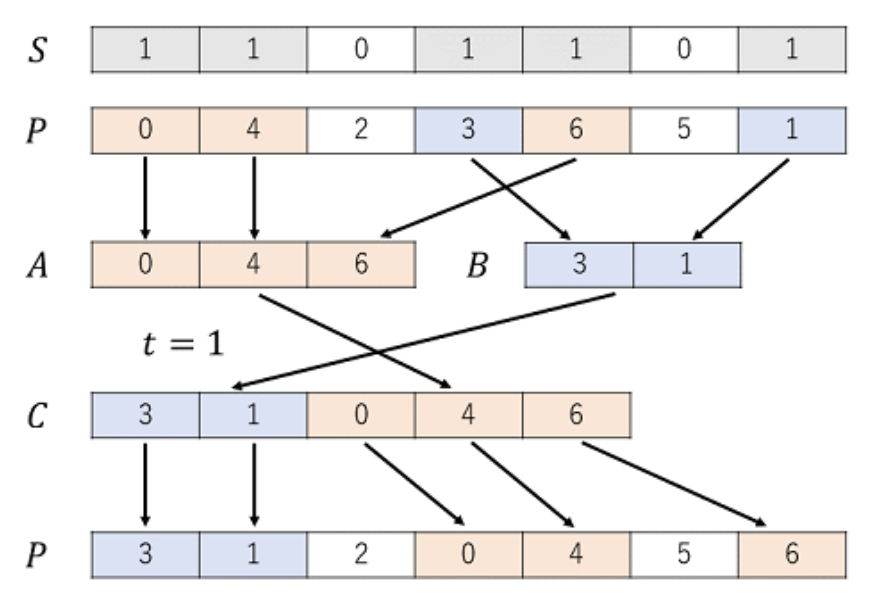### 「Petrozavodsk Summer 2020」Parity Sort# 「Petrozavodsk Summer 2020」Parity Sort

1. 有两个空序列 $A$ 和 $B$
2. 枚举 $i$ 从 $1$ 到 $n$
• 如果 $S_i=0$，不进行操作
• 如果 $S_i=1$，如果 $P_i$ 是偶数，则放到 $A$ 的末尾，否则放到 $B$ 的末尾
3. 如果 $t=0$，$C=\overline{AB}$；否则 $C=\overline{BA}$。
4. 枚举 $i$ 从 $1$ 到 $n$
• 如果 $S_i=0$，不进行操作
• 如果 $S_i=1$，将 $P_i$ 设为 $C$ 的开头元素，删去 $C$ 的开头元素

$1\le n\le 15000$。

## 题意补充